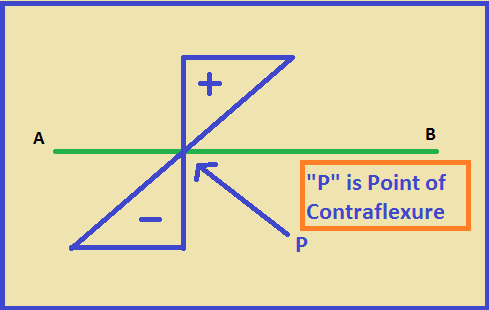# Point of contraflexure – Zero bending moment at a section of Beam

Point of contraflexure is the point at which bending moment is zero i.e the bending moment changes its sign.

It means that the value of the bending moment changes its sign from negative to positive or positive to negative.

Also, We can say at that point the zone of compression and tension are separated.

While designing any structure we have to know about the required amount of bending moment in structure. So, that we can provide the required bending moment at any section of the structure.

If there is zero bending moment at any section then there will be no worth of providing concrete or steel rod to be expensive and increase the dead load.

Let us know from the figure of beam below.

## Point of ContraflexurePoint of contraflexure – Zero bending moment at a section of Beam

From the figure, “AB” is a beam where the bending moment has some value. But when we talk about point “P” then the bending moment does not have any value.

It means it has zero value. After this point, the bending moment has a positive value. So, this point of the beam is known as Point of contraflexure.

Again let us take another example.

Look at this picture, The diagram does not have a negative bending moment. It has only a positive bending moment whose values are only in an upward direction.

So, It does not have Point of Contraflexure.

Shear Force Diagram and Bending Moment Diagram

Easy way to calculate unit weight of steel rod | 6 Types of steel reinforcement

Moment of inertia formula | Definition for moment of inertia

100% Free online AutoCAD course-Basic to Advance

### 1 thought on “Point of contraflexure – Zero bending moment at a section of Beam”

1.I like it!